# TL;DR

• Four Decades of Free and Open Source Software, collaboration between industry and academia: high performance linear algebra (netlib), available in Scala (Breeze).
• Not Invented Here: lukewarm performance, poor precision, dangerous results.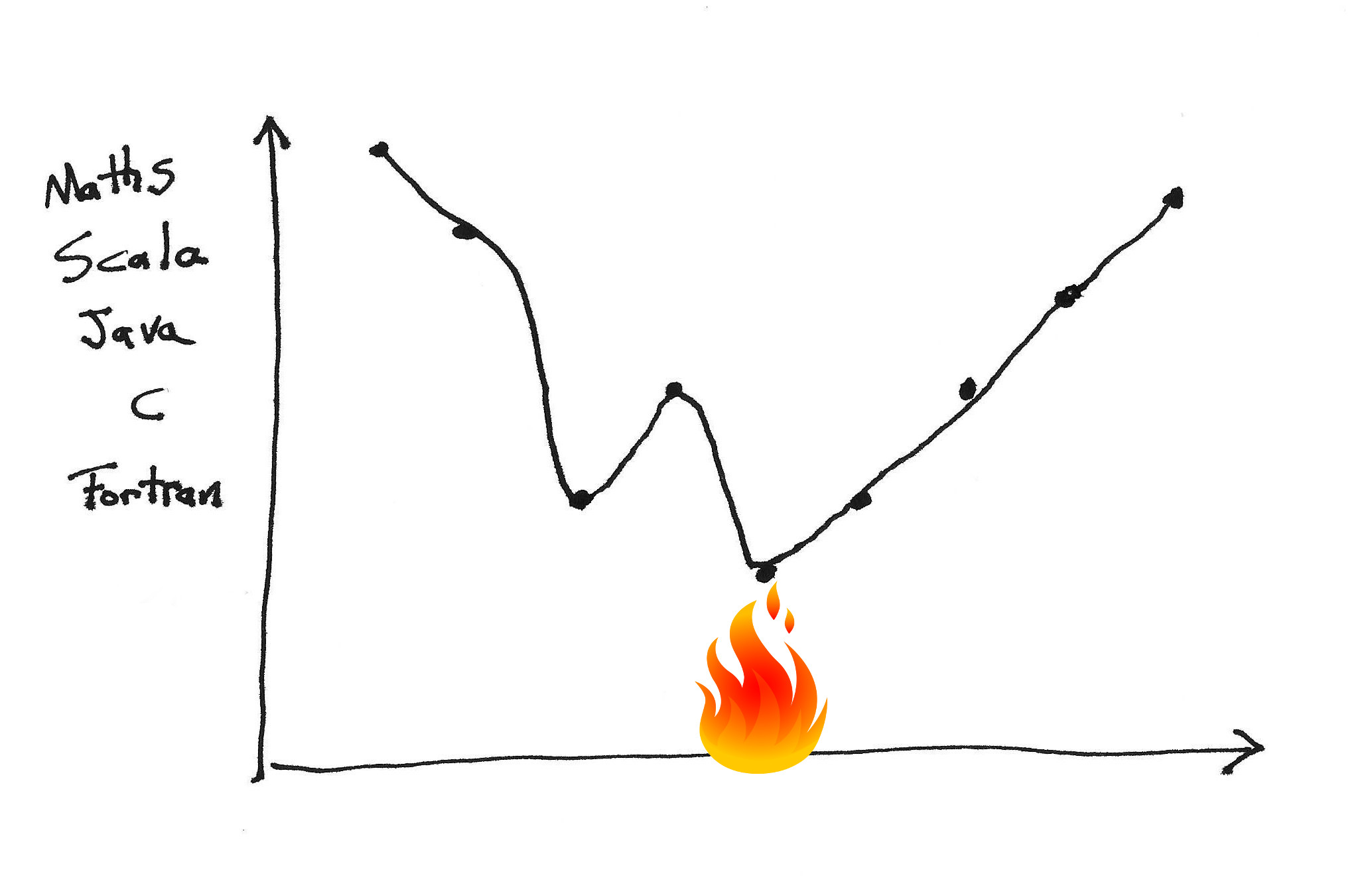# Definition

• A Ring is a set with multiplication and addition.
• A Field is a ring where multiplication is commutative ($$a.b = b.a$$) and nonzero elements have an inverse ($$a.a^{-1} = 1$$).
• A Vector Space has elements from a Field (scalars) and a set (vectors) with vector addition ($$v + m$$) and scaling ($$\alpha . b$$)
• A Basis of vectors $${v_1, v_2, \ldots, v_n}$$ defines a coordinate system under which all other vectors can be represented: $$a_1 v_1 + a_2 v_2 + \ldots + a_n v_n$$
• A Matrix encodes mappings between bases# Diagonalisation

• $$D = P^{-1} A P$$
• $$D_{ij} = \delta_{ij}\lambda_i$$
• $$AP = DP$$
• $$A v_k = \lambda v_k$$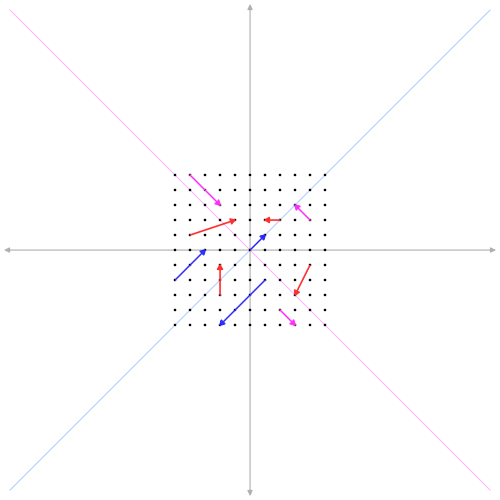# Example: Kalman Filter$\mathbf{x}_{k} = \mathbf{F}_{k} \mathbf{x}_{k-1} + \mathbf{B}_{k} \mathbf{u}_{k} + \mathbf{w}_{k}$

• $$\mathbf{x}_k$$ is the state of the system at time slice $$k$$
• $$\mathbf{F}_k$$ is the state transition model
• $$\mathbf{B}_k$$ is the control-input model (applied to control vector $$\mathbf{u}_k$$)
• $$\mathbf{w}_k$$ is the process noise, covariance $$\mathbf{Q}_k$$
• observations $$\mathbf{z}_k$$ are made of the true state $$\mathbf{x}_k$$ according to
• $$\mathbf{z}_k = \mathbf{H}_{k} \mathbf{x}_k + \mathbf{v}_k$$
• $$\mathbf{H}_k$$ is the observation model and $$\mathbf{v}_k$$ is the observation noise (covariance $$\mathbf{R}_k$$)

# Example: PCA• $$\sigma_A^2 = \frac 1 n \sum_i^n a_i^2$$
• $$\sigma_B^2 = \frac 1 n \sum_i^n b_i^2$$
• $$\sigma_{AB}^2 = \frac 1 n \sum_i^n a_i b_i = \frac 1 n a b^T$$
• Data Matrix $$X$$
• all samples for one type of measurement per row
• different measure in each column
• all entries are diffs to the mean vector
• $$C_x = \frac 1 n X X^T$$
• $$Y = PX$$
• $$C_y = P C_x P^T$$
• find $$P$$ such that:
• $$C_{ii}$$ is maximised
• $$C_{ij}$$ is minimised $$i \neq j$$

$\begin{eqnarray*} C_y &=& P C_x P^T \\ &=& P P^{-1} D P P^T \\ &=& D \end{eqnarray*}$

# SVD

• $$X = U \Sigma V^T$$
• $$U$$ is orthogonal (rotation)
• $$\Sigma$$ is diagonal (stretch)
• $$V$$ is orthogonal (rotation)
• $$XX^T = (U \Sigma V^T) (U \Sigma V^T)^T = U \Sigma^2 U^T$$
• $$D^2 = C_y^2 = \Sigma$$
• $$U = P$$ (recall $$Y = PX$$)

# Multiplication

$A=\begin{pmatrix} A_{11} & A_{12} & \cdots & A_{1m} \\ A_{21} & A_{22} & \cdots & A_{2m} \\ \vdots & \vdots & \ddots & \vdots \\ A_{n1} & A_{n2} & \cdots & A_{nm} \\ \end{pmatrix} \qquad B=\begin{pmatrix} B_{11} & B_{12} & \cdots & B_{1p} \\ B_{21} & B_{22} & \cdots & B_{2p} \\ \vdots & \vdots & \ddots & \vdots \\ B_{m1} & B_{m2} & \cdots & B_{mp} \\ \end{pmatrix}$

$(A B)_{ij} = \sum_{k=1}^m A_{ik}B_{kj}$

$\small \begin{pmatrix} \boldsymbol a & \boldsymbol b & \boldsymbol c \\ . & . & . \\ . & . & . \\ \end{pmatrix} \begin{pmatrix} \boldsymbol r & . & . \\ \boldsymbol u & . & . \\ \boldsymbol x & . & . \\ \end{pmatrix} = \begin{pmatrix} \boldsymbol \alpha & . & . \\ . & . & . \\ . & . & . \\ \end{pmatrix} \\[25pt] \begin{pmatrix} . & . & . \\ \boldsymbol d & \boldsymbol e & \boldsymbol f \\ . & . & . \\ \end{pmatrix} \begin{pmatrix} \boldsymbol r & . & . \\ \boldsymbol u & . & . \\ \boldsymbol x & . & . \\ \end{pmatrix} = \begin{pmatrix} . & . & . \\ \boldsymbol \delta & . & . \\ . & . & . \\ \end{pmatrix} \\[25pt] \begin{pmatrix} . & . & . \\ \boldsymbol d & \boldsymbol e & \boldsymbol f \\ . & . & . \\ \end{pmatrix} \begin{pmatrix} . & \boldsymbol s & . \\ . & \boldsymbol v & . \\ . & \boldsymbol y & . \\ \end{pmatrix} = \begin{pmatrix} . & . & . \\ . & \boldsymbol \epsilon & . \\ . & . & . \\ \end{pmatrix}$

# Immutable

  case class ImmutableMatrix(
numRows: Int,
numCols: Int,
data: Vector[Vector[Double]]
) {
def mult(that: ImmutableMatrix): ImmutableMatrix = {
require(numCols == that.numRows)
val res = Array.fill(numRows, that.numCols)(0.0)
for {
i <- 0 until numRows
j <- 0 until that.numCols
k <- 0 until numCols
} {
res(i)(j) = data(i)(k) * that.data(k)(j)
}
ImmutableMatrix(numRows, numCols, ImmutableMatrix.arrayToVec(res))
}
}

# Naive

  trait Matrix {
def numRows: Int
def numCols: Int
def set(row: Int, col: Int, value: Double): Unit
def get(row: Int, col: Int): Double
def mult(that: Matrix): Matrix
}

class NaiveMatrix (
val numRows: Int,
val numCols: Int,
val values: Array[Array[Double]]
) extends Matrix {

def set(row: Int, col: Int, value: Double): Unit =
values(row)(col) = value

def get(row: Int, col: Int): Double = values(row)(col)
    def mult(that: Matrix): Matrix = {
val res = NaiveMatrix(numRows, that.numCols)
for {
i <- 0 until numRows
j <- 0 until that.numCols
k <- 0 until numCols
} {
val update = this.get(i, k) * that.get(k, j)
res.set(i, j, res.get(i, j) + update)
}
res
}
}

# Naive Parallel

  trait NaiveParallelMatrix {
this: NaiveMatrix =>
override def mult(that: Matrix): Matrix = {
val res = NaiveMatrix(numRows, that.numCols)
for {
i <- (0 until numRows).par
j <- (0 until that.numCols).par
k <- 0 until numCols
} {
val update = this.get(i, k) * that.get(k, j)
res.set(i, j, res.get(i, j) + update)
}
res
}
}

# Allocationhttp://github.com/fommil/lions-share

# While Loops

  trait NaiveWhileMatrix {
this: NaiveMatrix =>
override def mult(that: Matrix): Matrix = {
val res = NaiveMatrix(numRows, that.numCols)
var i, j, k = 0
while (i < numRows) {
j = 0
while (j < that.numCols) {
k = 0
var sum = 0.0
while (k < numCols) {
sum += this.get(i, k) * that.get(k, j)
k += 1
}
res.set(i, j, sum)
j += 1
}
i += 1
}
res
}
}

# Comparison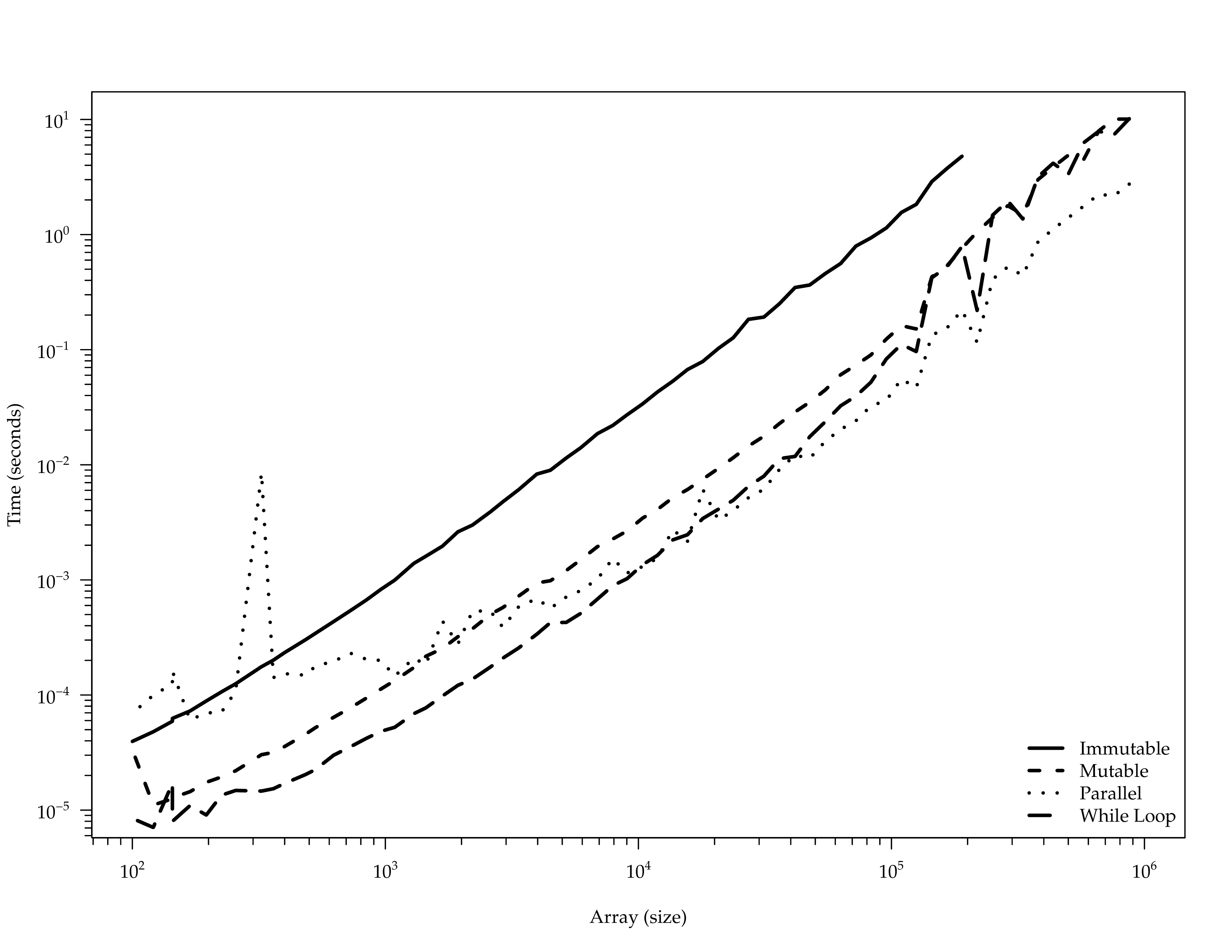# netlib.org# BLAS

Level Name Operation
1 xAXPY $$y \leftarrow \alpha x + y$$
1 xDOT $$dot \leftarrow x^T y$$
1 ...
2 xGEMV $$y \leftarrow \alpha A x + \beta y$$
2 xTRSV $$T \boldsymbol x = y$$
2 ...
3 xGEMM $$C \leftarrow \alpha A B + \beta C$$
3 xTRSM $$T \boldsymbol B = \alpha \boldsymbol B$$
3 ...

# Reference DGEMM

   *         Form  C := alpha*A*B + beta*C
DO 90 J = 1,N
IF (BETA.EQ.ZERO) THEN
DO 50 I = 1,M
C(I,J) = ZERO
50                 CONTINUE
ELSE IF (BETA.NE.ONE) THEN
DO 60 I = 1,M
C(I,J) = BETA*C(I,J)
60                 CONTINUE
END IF
DO 80 L = 1,K
IF (B(L,J).NE.ZERO) THEN
TEMP = ALPHA*B(L,J)
DO 70 I = 1,M
C(I,J) = C(I,J) + TEMP*A(I,L)
70                     CONTINUE
END IF
80             CONTINUE
90         CONTINUE

# LAPACK

dbdsdc dbdsqr ddisna dgbbrd dgbcon dgbequ dgbequb dgbrfs dgbrfsx dgbsv
dgbsvx dgbsvxx dgbtrf dgbtrs dgebak dgebal dgebrd dgecon dgeequ
dgeequb dgees dgeesx dgeev dgeevx dgehrd dgejsv dgelqf dgels dgelsd
dgelss dgelsy dgeqlf dgeqp3 dgeqpf dgeqrf dgeqrfp dgerfs dgerfsx
dgerqf dgesdd dgesv dgesvd dgesvj dgesvx dgesvxx dgetrf dgetri dgetrs
dggbak dggbal dgges dggesx dggev dggevx dggglm dgghrd dgglse dggqrf
dggrqf dggsvd dggsvp dgtcon dgtrfs dgtsv dgtsvx dgttrf dgttrs dhgeqz
dhsein dhseqr dopgtr dopmtr dorgbr dorghr dorglq dorgql dorgqr dorgrq
dorgtr dormbr dormhr dormlq dormql dormqr dormrq dormrz dormtr dpbcon
dpbequ dpbrfs dpbstf dpbsv dpbsvx dpbtrf dpbtrs dpftrf dpftri dpftrs
dpocon dpoequ dpoequb dporfs dporfsx dposv dposvx dposvxx dpotrf
dpotri dpotrs dppcon dppequ dpprfs dppsv dppsvx dpptrf dpptri dpptrs
dpstrf dptcon dpteqr dptrfs dptsv dptsvx dpttrf dpttrs dsbev dsbevd
dsbevx dsbgst dsbgv dsbgvd dsbgvx dsbtrd dsfrk dspcon dspev dspevd
dspevx dspgst dspgv dspgvd dspgvx dsprfs dspsv dspsvx dsptrd dsptrf
dsptri dsptrs dstebz dstedc dstegr dstein dstemr dsteqr dsterf dstev
dstevd dstevr dstevx dsycon dsyequb dsyev dsyevd dsyevr dsyevx dsygst
dsygv dsygvd dsygvx dsyrfs dsyrfsx dsysv dsysvx dsysvxx dsytrd dsytrf
dsytri dsytrs dtbcon dtbrfs dtbtrs dtfsm dtftri dtfttp dtfttr dtgevc
dtgexc dtgsen dtgsja dtgsna dtgsyl dtpcon dtprfs dtptri dtptrs dtpttf
dtpttr dtrcon dtrevc dtrexc dtrrfs dtrsen dtrsna dtrsyl dtrtri dtrtrs
dtrttf dtrttp dtzrzf dsgesv dsposv


# DGETRF

$LP^{-1}U = A$# Optional: Solving with LU

• $$Ax=b$$
• $$PA = LU$$
• $$LUx = Pb$$
1. $$Ly = Pb$$
2. $$Ux = y$$

$\begin{matrix} l_{1,1} x_1 & & & & & = & b_1 \\ l_{2,1} x_1 & + & l_{2,2} x_2 & & & = & b_2 \\ \vdots & & \vdots & \ddots & & & \vdots \\ l_{m,1} x_1 & + & l_{m,2} x_2 & + \dotsb + & l_{m,m} x_m & = & b_m \\ \end{matrix}$

$x_1 = \frac{b_1}{l_{1,1}} \\ x_2 = \frac{b_2 - l_{2,1} x_1}{l_{2,2}} \\ x_m = \frac{b_m - \sum_{i=1}^{m-1} l_{m,i}x_i}{l_{m,m}} \\$

# C API

#include "cblas.h"

void cblas_dgemm(const enum CBLAS_ORDER Order, const enum CBLAS_TRANSPOSE TransA,
const enum CBLAS_TRANSPOSE TransB, const int M, const int N,
const int K, const double alpha, const double  *A,
const int lda, const double  *B, const int ldb,
const double beta, double  *C, const int ldc) {
...
if( Order == CblasColMajor ) {
if(TransA == CblasTrans) TA='T';
else if ( TransA == CblasConjTrans ) TA='C';
else if ( TransA == CblasNoTrans )   TA='N';
else ...

if(TransB == CblasTrans) TB='T';
else if ( TransB == CblasConjTrans ) TB='C';
else if ( TransB == CblasNoTrans )   TB='N';
else ...

#ifdef F77_CHAR
F77_TA = C2F_CHAR(&TA);
F77_TB = C2F_CHAR(&TB);
#endif

F77_dgemm(F77_TA, F77_TB, &F77_M, &F77_N, &F77_K, &alpha, A,
&F77_lda, B, &F77_ldb, &beta, C, &F77_ldc);
} else if (Order == CblasRowMajor) { ...

# Kinds of Error

• roundoff error
• input error
• machine precision: $$\epsilon$$

# Types of Structures

• Scalar, numbers, e.g. an eigenvalue
• Vector, e.g. the solution $$x$$ of system $$Ax=b$$
• Matrix, e.g. matrix inverse $$A^{-1}$$
• Subspace, e.g. space spanned by eigenvectors

# Relative Errors

• $$\hat{\alpha} \approx \alpha$$
• $$\vert \hat{\alpha} - \alpha \vert$$
• $$\vert \hat{\alpha} - \alpha \vert / \vert \alpha \vert$$
• $$\vert \hat{\alpha} - \alpha \vert / \vert \hat{\alpha} \vert$$
• $$10^{-5}\epsilon$$ "5 decimal digits"

# Norms

Name Vector Matrix
1-norm $$\Vert x\Vert _{1} = \sum_i \vert x_i\vert$$ $$\Vert A\Vert _{1} = \max_j \sum_i \vert a_{ij}\vert$$
2-norm $$\Vert x\Vert _2 = ( \sum_i \vert x_i\vert^2 )^{1/2}$$ $$\Vert A\Vert _2 = \max_{x \neq 0} \Vert Ax\Vert _2 / \Vert x\Vert _2$$
Frobenius $$\Vert x \Vert_F = \Vert x \Vert_2$$ $$\Vert A\Vert _F = ( \sum_{ij} \vert a_{ij}\vert^2 )^{1/2}$$
$$\infty$$ norm $$\Vert x\Vert _{\infty} = \max_i \vert x_i\vert$$ $$\Vert A\Vert _{\infty} = \max_i \sum_j \vert a_{ij}\vert$$

# Condition Number

$A = \left( \begin{array}{ccc} 1 & 2 & 3 \\ 4 & 5 & 6 \\ 7 & 8 & 10 \end{array} \right) \qquad A^{-1} \approx \left( \begin{array}{ccc} -.667 & -1.333 & 1 \\ -.667 & 3.667 & -2 \\ 1 & -2 & 1 \end{array} \right)$

$\kappa_{\infty}(A) = 158.33$

LAPACK RCOND(A) $$\approx \frac 1 {\kappa_\infty}$$

# Example

$Ax = b \\ A = \left( \begin{array}{ccc} 4 & 16000 & 17000 \\ 2 & 5 & 8 \\ 3 & 6 & 10 \end{array}\right) \qquad b = \left( \begin{array}{c} 100.1 \\ .1 \\ .01 \end{array}\right)$

ANORM = SLANGE( 'I', N, N, A, LDA, WORK )
CALL SGESV( N, 1, A, LDA, IPIV, B, LDB, INFO )
CALL SGECON( 'I', N, A, LDA, ANORM, RCOND, WORK, IWORK, INFO )
ERRBD = EPSMCH / RCOND
• SGESV ERRBD $$= 1.5\cdot 10^{-2}$$
• true error $$= 1.5\cdot 10^{-3}$$
• SGESVX FERR $$= 3.0 \cdot 10^{-5}$$
• true error $$4.3 \cdot 10^{-7}$$

# Hardware Support

$$y = a * x + b$$

Mnemonic Operands Operation
VFMADD132SD xmm,xmm,xmm/m64 $0=$0×$2+$1
VFMADD213SD xmm,xmm,xmm/m64 $0=$1×$0+$2
VFMADD231SD xmm,xmm,xmm/m64 $0=$1×$2+$0

# Bottleneck

    requestStart = currentTimeNanos();
double* b = calloc(length, sizeof(double));

for (i = 0 ; i < m ; i++) {
c = i * m;
for (j = 0 ; j < m ; j ++) {
for (k = 0 ; k < m ; k++) {
tmp = a[c + k];
}
b[c + j] = tmp;
#else
b[c + j] = a[c + j];
#endif
}
}
requestEnd = currentTimeNanos();

# Timings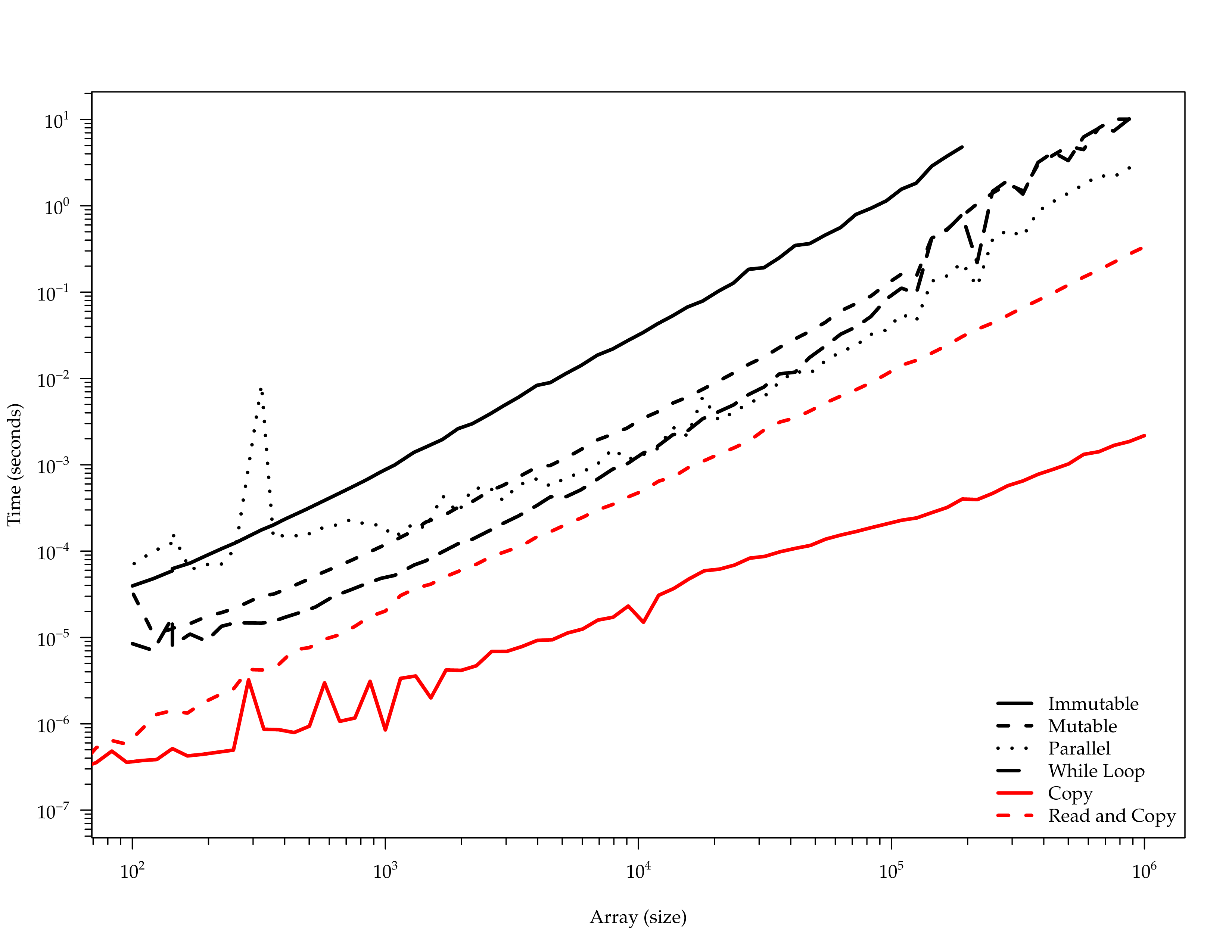# CPU Architecture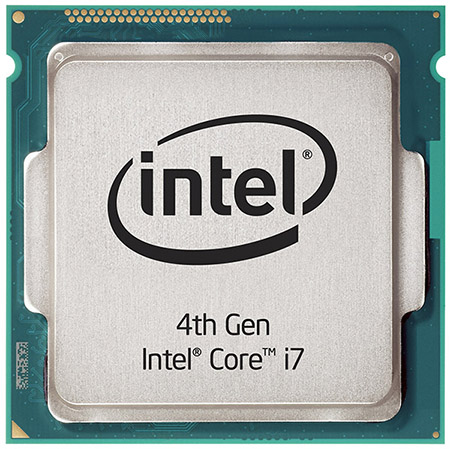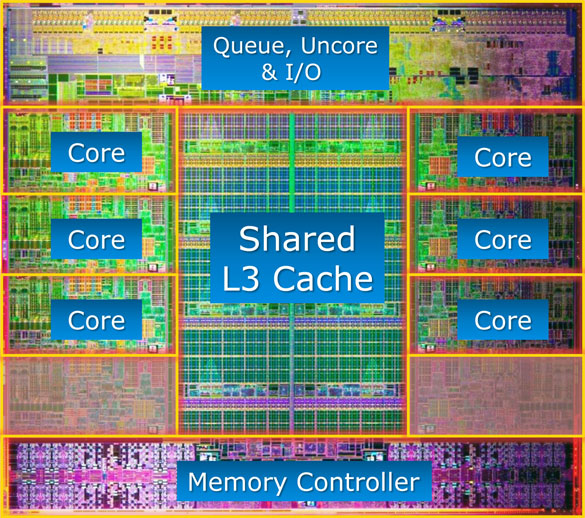# CPU Cycles

80287 80 120 200 180
80387 30 45 90 125
80486 15 16 73 85
Pentium 2 2 39 70
Pentium II 2 4 25 35
Pentium 4 3 5 30 30
... ... ... ... ...
i7 $$\frac 1 {32}$$ $$\frac 1 {32}$$ $$\frac 1 {32}$$ $$\frac 1 {32}$$

# Latency

Computer Human Timescale Human Analogy
L1 cache reference 0.5 secs One heart beat
Branch mispredict 5 secs Yawn
L2 cache reference 7 secs Long yawn
Mutex lock/unlock 25 secs Making a cup of tea
Main memory reference 100 secs Brushing your teeth
Compress 1K bytes with Zippy 50 min Scala compiler CI pipeline
Send 2K bytes over 1Gbps network 5.5 hr Train London to Edinburgh
SSD random read 1.7 days Weekend
Read 1MB sequentially from memory 2.9 days Long weekend
Round trip within same datacenter 5.8 days Short holiday
Read 1MB sequentially from SSD 11.6 days Holiday
Disk seek 16.5 weeks Term of university
Read 1MB sequentially from disk 7.8 months Fully paid maternity in Norway
Send packet CA->Netherlands->CA 4.8 years Government's term

# Intel

### Parallel Studio

Cluster Professional Composer
Named User $2,949 ($1,049) $2,299 ($799) $1,449 ($499)
2 Floating Users $14,749 ($5,199) $11,499 ($4,049) $5,099 ($1,799)
5 Floating Users $29,499 ($10,349) $22,999 ($8,049) $10,899 ($3,849)

$499 # AMD Core Math Library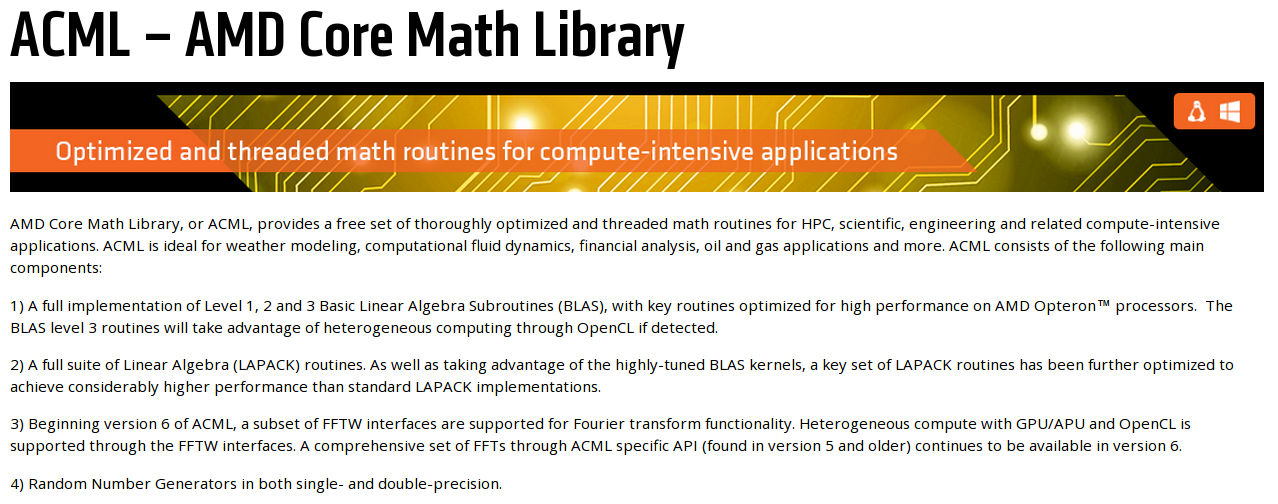# Apple vecLib / Accelerate $ ll /usr/lib/lib{blas, lapack}.dylib
/usr/lib/libblas.dylib -> /System/Library/Frameworks/vecLib.framework/Versions/A/libBLAS.dylib
/usr/lib/liblapack.dylib -> /System/Library/Frameworks/vecLib.framework/Versions/A/libLAPACK.dylib

$tree /System/Library/Frameworks/vecLib.framework/Versions/Current/ ├── _CodeSignature │ └── CodeResources ├── libBLAS.dylib ├── libLAPACK.dylib ├── libvDSP.dylib ├── libvMisc.dylib ├── Resources │ ├── English.lproj │ │ └── InfoPlist.strings │ ├── Info.plist │ └── version.plist └── vecLib  # ATLAS • compiler flags - free wins • parameterisation • cache edges - how much to attempt in kernels? • copy - matrix data can be rearranged optimally • multiple implementations of the same function • Fred's loop is faster than Bob's on your machine • code generation • inline on steroids cat /usr/share/doc/libatlas3-base/README.Debian for A in /sys/devices/system/cpu/cpu*/cpufreq/scaling_governor do sudo sh -c "echo performance >$A"
done
cat /sys/devices/system/cpu/cpu*/cpufreq/scaling_governor

sudo apt-get source atlas
sudo apt-get build-dep atlas
sudo apt-get install devscripts
cd atlas-*
fakeroot debian/rules custom
sudo dpkg -i ../libatlas*.deb

sudo update-alternatives --config libblas.so.3
sudo update-alternatives --config liblapack.so.3


$ls OpenBLAS/kernel/x86_64/*dgemm* dgemm_kernel_16x2_haswell.S dgemm_kernel_6x4_piledriver.S dgemm_ncopy_2.S dgemm_ncopy_8.S dgemm_tcopy_8_bulldozer.S dgemm_kernel_4x4_haswell.S dgemm_kernel_8x2_bulldozer.S dgemm_ncopy_4.S dgemm_tcopy_2.S dgemm_tcopy_8.S dgemm_kernel_4x8_sandy.S dgemm_kernel_8x2_piledriver.S dgemm_ncopy_8_bulldozer.S dgemm_tcopy_4.S  # DGEMM Benchmarks requestStart = currentTimeNanos(); double* c = calloc(m * m, sizeof(double)); cblas_dgemm(CblasColMajor, CblasNoTrans, CblasNoTrans, m, m, m, 1, a, m, b, m, 0, c, m); requestEnd = currentTimeNanos(); LD_LIBRARY_PATH=. ./dgemmtest > ../R/netlib-ref.csv LD_LIBRARY_PATH=/usr/lib/atlas-base ./dgemmtest > ../R/atlas.csv LD_LIBRARY_PATH=/usr/lib/openblas-base ./dgemmtest > ../R/openblas.csv LD_LIBRARY_PATH=/opt/acml5.3.1/gfortran64_mp/lib/:. ./dgemmtest > ../R/acml.csv LD_LIBRARY_PATH=/opt/intel/mkl/lib/intel64:. ./dgemmtest > ../R/intel.csv  # DGEMM Comparison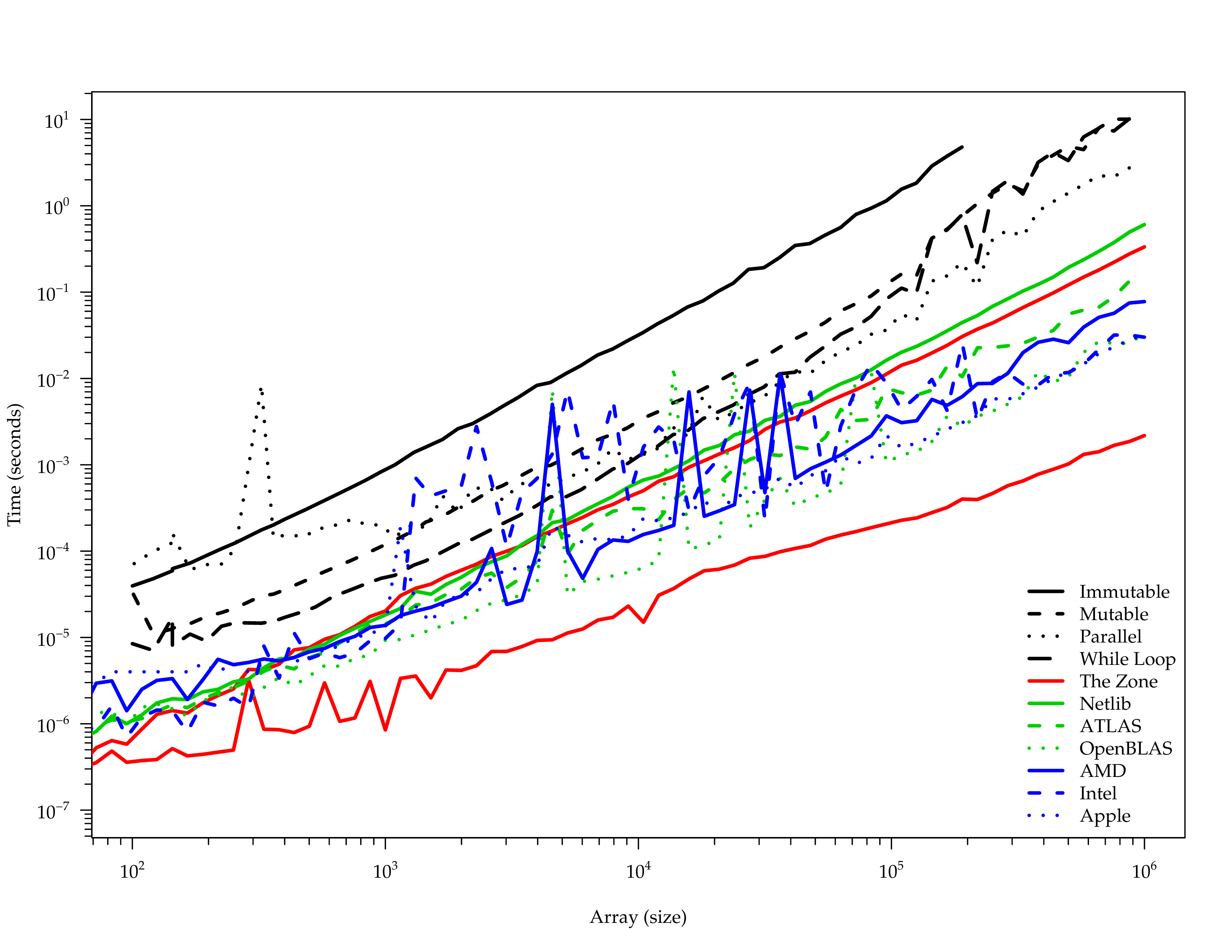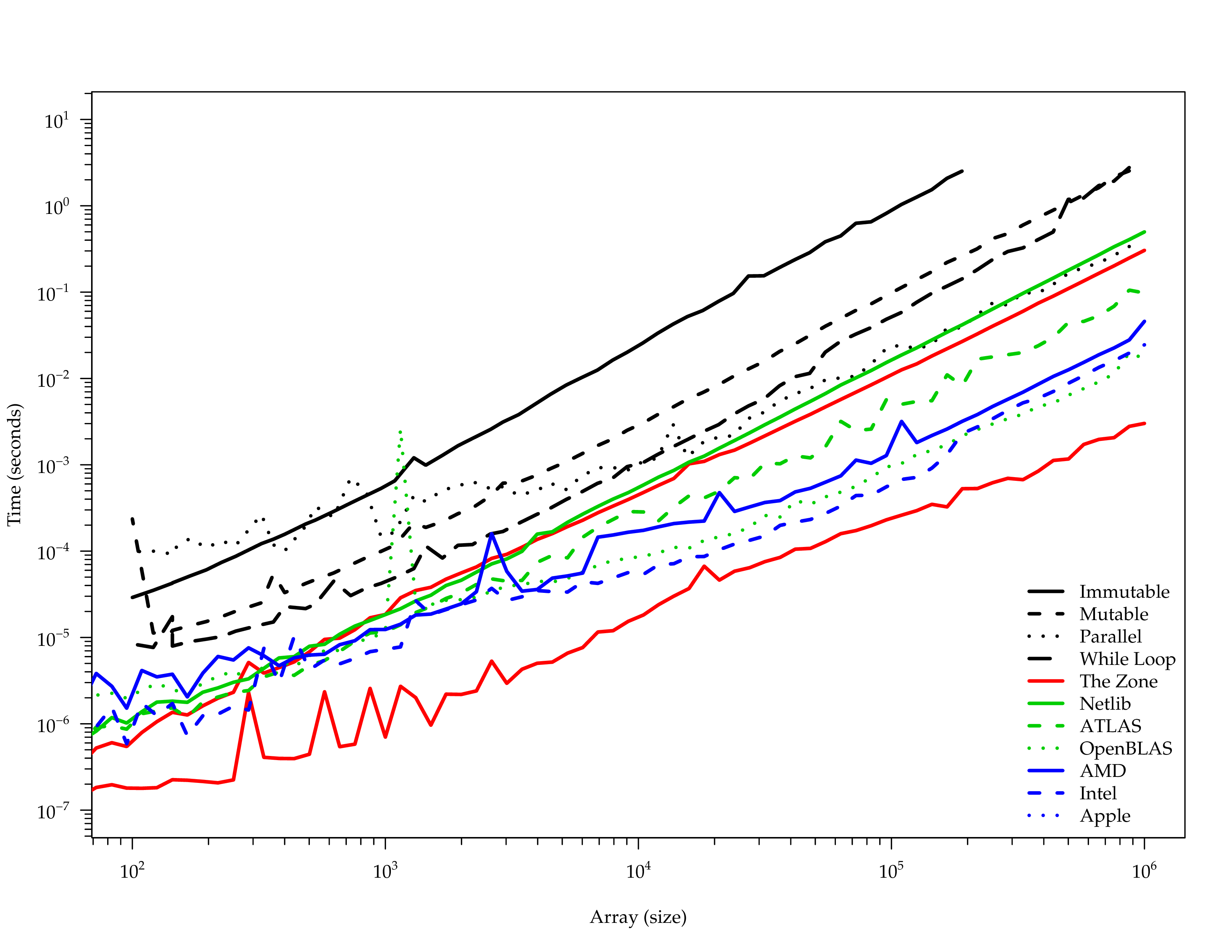# netlib-java # Fortran to ...  IF( UPPER )THEN DO 70, J = 1, N DO 60, I = 1, M TEMP1 = ALPHA*B( I, J ) TEMP2 = ZERO DO 50, K = 1, I - 1 C( K, J ) = C( K, J ) + TEMP1 *A( K, I ) TEMP2 = TEMP2 + B( K, J )*A( K, I ) 50 CONTINUE IF( BETA.EQ.ZERO )THEN C( I, J ) = TEMP1*A( I, I ) + ALPHA*TEMP2 ELSE C( I, J ) = BETA *C( I, J ) +$                           TEMP1*A( I, I ) + ALPHA*TEMP2
END IF
60          CONTINUE
70       CONTINUE

# ... Java

if (upper) {
forloop70: for (j = 1; j <= n; j++) {
forloop60: for (i = 1; i <= m; i++) {
temp1 = alpha * b[(i) - 1 + (j - 1) * ldb + _b_offset];
temp2 = zero;
forloop50: for (k = 1; k <= i - 1; k++) {
c[(k) - 1 + (j - 1) * Ldc + _c_offset] = c[(k) - 1 + (j - 1) * Ldc + _c_offset] + temp1 * a[(k) - 1 + (i - 1) * lda + _a_offset];
temp2 = temp2 + b[(k) - 1 + (j - 1) * ldb + _b_offset] * a[(k) - 1 + (i - 1) * lda + _a_offset];
Dummy.label("Dsymm", 50);
}
if (beta == zero) {
c[(i) - 1 + (j - 1) * Ldc + _c_offset] = temp1 * a[(i) - 1 + (i - 1) * lda + _a_offset] + alpha * temp2;
} else {
c[(i) - 1 + (j - 1) * Ldc + _c_offset] = beta * c[(i) - 1 + (j - 1) * Ldc + _c_offset] + temp1 * a[(i) - 1 + (i - 1) * lda + _a_offset] + alpha * temp2;
}
Dummy.label("Dsymm", 60);
}
Dummy.label("Dsymm", 70);
}
}

# API Generator

abstract class BLAS {
...
/**
* ... original docs here ...
*/
abstract public void dgemm(
String transa,
String transb,
int m,
int n,
int k,
double alpha,
double[] a,
int lda,
double[] b,
int ldb,
double beta,
double[] c,
int Ldc
);
...
}

# Regex Digression

StringBuilder regex = new StringBuilder();
regex.append(format(">\\Q%s\\E</A></(?:B|strong)>\$$", name)); for (Class klass : types) { regex.append(format( ",?\\s*(?:<A[^>]+>)?[\\w.]*\\Q%s\\E(?:</A>)?(?:&lt;[^&]+&gt;)?&nbsp;([^),\\s]+)", klass.getSimpleName() )); } regex.append(format("\$$</CODE>"));

Pattern pattern = Pattern.compile(regex.toString(), MULTILINE | CASE_INSENSITIVE);
Matcher matcher = pattern.matcher(raw);

# Implementations

### F2jBLAS

@Override
public void dgemm(
String transa, String transb, int m, int n, int k,
double alpha, double[] a, int lda,
double[] b, int ldb, double beta, double[] c, int Ldc
) {
org.netlib.blas.Dgemm.dgemm(
transa, transb, m, n, k, alpha, a, 0, lda, b, 0, ldb, beta, c, 0, Ldc
);
}

### NativeRefBLAS and NativeSystemBLAS

@Override
public native void dgemm(
String transa, String transb, int m, int n, int k,
double alpha, double[] a, int lda,
double[] b, int ldb, double beta,
double[] c, int Ldc
);

# JNI

JNIEXPORT void JNICALL Java_com_github_fommil_netlib_NativeSystemBLAS_dgemm (JNIEnv * env, jobject calling_obj, jstring transa, jstring transb, jint m, jint n, jint k, jdouble alpha, jdoubleArray a, jint lda, jdoubleArray b, jint ldb, jdouble beta, jdoubleArray c, jint Ldc) {
char * jni_transa = (char *)(*env)->GetStringUTFChars(env, transa, JNI_FALSE);
char * jni_transb = (char *)(*env)->GetStringUTFChars(env, transb, JNI_FALSE);
jdouble * jni_a = NULL;
if (a != NULL) {
jni_a = (*env)->GetPrimitiveArrayCritical(env, a, JNI_FALSE);
check_memory(env, jni_a);
}
jdouble * jni_b = NULL;
if (b != NULL) {
jni_b = (*env)->GetPrimitiveArrayCritical(env, b, JNI_FALSE);
check_memory(env, jni_b);
}
jdouble * jni_c = NULL;
if (c != NULL) {
jni_c = (*env)->GetPrimitiveArrayCritical(env, c, JNI_FALSE);
check_memory(env, jni_c);
}
cblas_dgemm(CblasColMajor, getCblasTrans(jni_transa), getCblasTrans(jni_transb), m, n, k, alpha, jni_a, lda, jni_b, ldb, beta, jni_c, Ldc);
if (c != NULL) (*env)->ReleasePrimitiveArrayCritical(env, c, jni_c, 0);
if (b != NULL) (*env)->ReleasePrimitiveArrayCritical(env, b, jni_b, 0);
if (a != NULL) (*env)->ReleasePrimitiveArrayCritical(env, a, jni_a, 0);
(*env)->ReleaseStringUTFChars(env, transb, jni_transb);
(*env)->ReleaseStringUTFChars(env, transa, jni_transa);
}

# Maven Native

<plugin>
<groupId>org.codehaus.mojo</groupId>
<artifactId>native-maven-plugin</artifactId>
<configuration>
<javahVerbose>true</javahVerbose>
<javahClassNames>
<javahClassName>com.github.fommil.netlib.NativeSystemBLAS</javahClassName>
</javahClassNames>
<compilerStartOptions>
<compilerStartOption>-O3</compilerStartOption>
</compilerStartOptions>
<sources>
<source>
<directory>${project.build.directory}/netlib-native</directory> <includes> <include>*.c</include> </includes> ... # JNILoader public class NativeSystemBLAS extends com.github.fommil.netlib.F2jBLAS { static { String jnilib = com.github.fommil.jni.JniNamer.getJniName("netlib-native_system"); String natives = System.getProperty("com.github.fommil.netlib.NativeSystemBLAS.natives", jnilib); com.github.fommil.jni.JniLoader.load(natives.split(",")); } ... } # Performance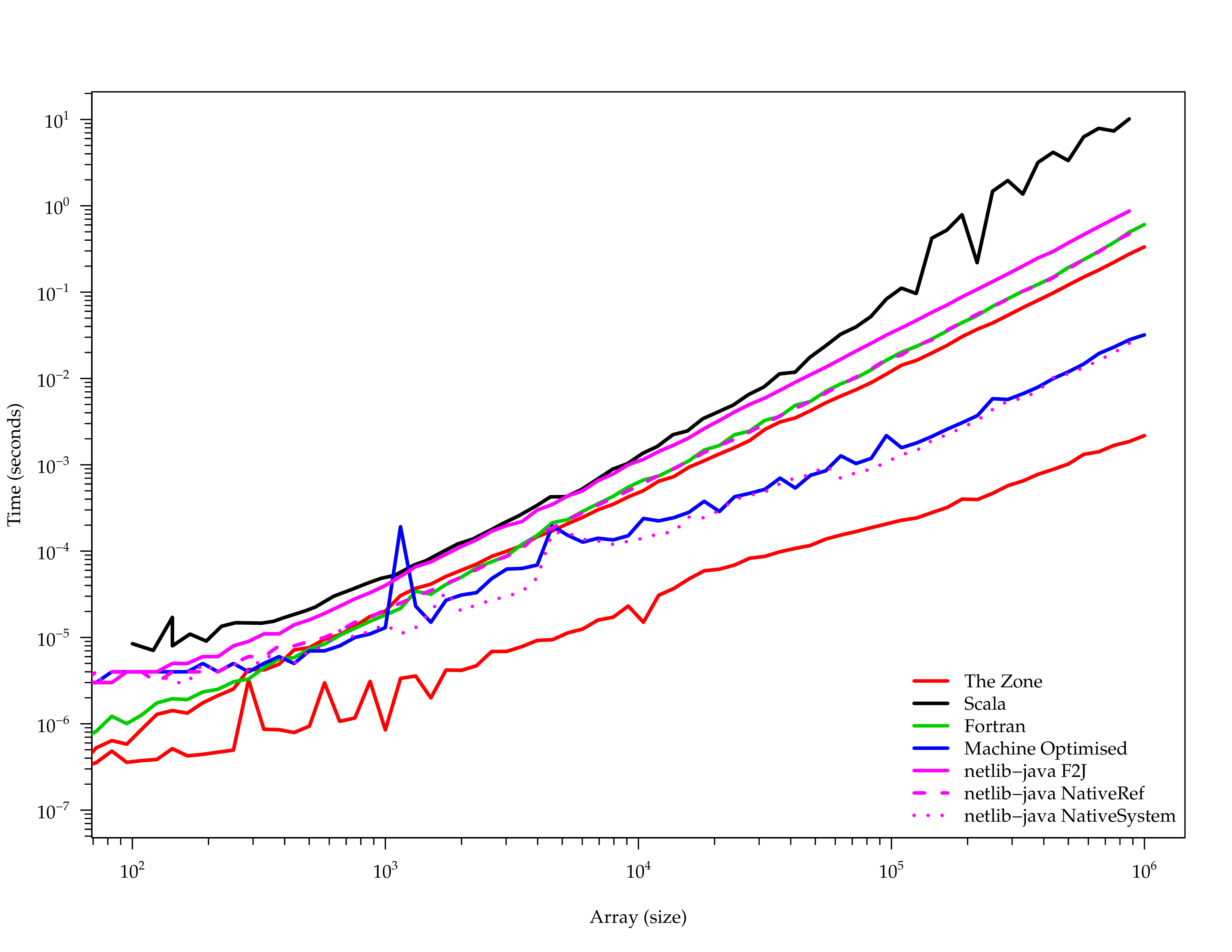# Scala API # Breeze libraryDependencies ++= Seq( "org.scalanlp" %% "breeze" % "0.10", "org.scalanlp" %% "breeze-natives" % "0.10" ) Nov 30, 2014 6:42:51 PM com.github.fommil.jni.JniLoader liberalLoad INFO: successfully loaded /tmp/jniloader7150057786941522144netlib-native_system-linux-x86_64.so  scala> import breeze.linalg._ scala> val x = DenseVector.zeros[Double](5) x: DenseVector[Double] = DenseVector(0.0, 0.0, 0.0, 0.0, 0.0) scala> x.t res1: Transpose[DenseVector[Double]] = Transpose(DenseVector(0.0, 0.0, 0.0, 0.0, 0.0)) scala> val m = DenseMatrix.zeros[Int](5,5) m: DenseMatrix[Int] = 0 0 0 0 0 0 0 0 0 0 0 0 0 0 0 0 0 0 0 0 0 0 0 0 0 scala> (m.rows, m.cols) (Int, Int) = (5,5) scala> m(::,1) DenseVector[Int] = DenseVector(0, 0, 0, 0, 0) scala> m(4,::) := DenseVector(1,2,3,4,5).t DenseMatrix[Int] = 1 2 3 4 5 scala> m DenseMatrix[Int] = 0 0 0 0 0 0 0 0 0 0 0 0 0 0 0 0 0 0 0 0 1 2 3 4 5 Operation Example Elementwise addition a + b Elementwise multiplication a :* b Vector dot product a dot b Elementwise sum sum(a) Elementwise max a.max Elementwise argmax argmax(a) Linear solve a b Transpose a.t Determinant det(a) Inverse inv(a) Pseudoinverse pinv(a) SVD SVD(u,s,v) = svd(a) # Example: Canon Ball  val dt = 0.1 val g = 9.8 def I = DenseMatrix.eye[Double](4) val B = DenseMatrix( (0.0, 0.0, 0.0, 0.0), (0.0, 0.0, 0.0, 0.0), (0.0, 0.0, -1.0, 0.0), (0.0, 0.0, 0.0, -1.0) ) val u = DenseVector(0, 0, g * Δt * Δt, g * Δt) val F = DenseMatrix( (1.0, dt, 0.0, 0.0), (0.0, 1.0, 0.0, 0.0), (0.0, 0.0, 1.0, dt), (0.0, 0.0, 0.0, 1.0) ) val H = I val Q = DenseMatrix.zeros[Double](4, 4) val R = I * 0.2 // guess of state and variance var s = DenseVector.zeros[Double](4) var P = I  while (x(2) >= 0) { // measurement def noisy(actual: Double) = actual + Random.nextGaussian * 50 val z = x.mapValues(noisy) // actual simulation x = F * x + B * u t += dt // prediction step val predS = F * s + B * u val predP = F * P * F.t + Q // observation step val innov = z - H * predS val innov_cov = H * predP * H.t + R // update step val gain = predP * H.t * inv(innov_cov) s = predS + gain * innov P = (I - gain * H) * predP }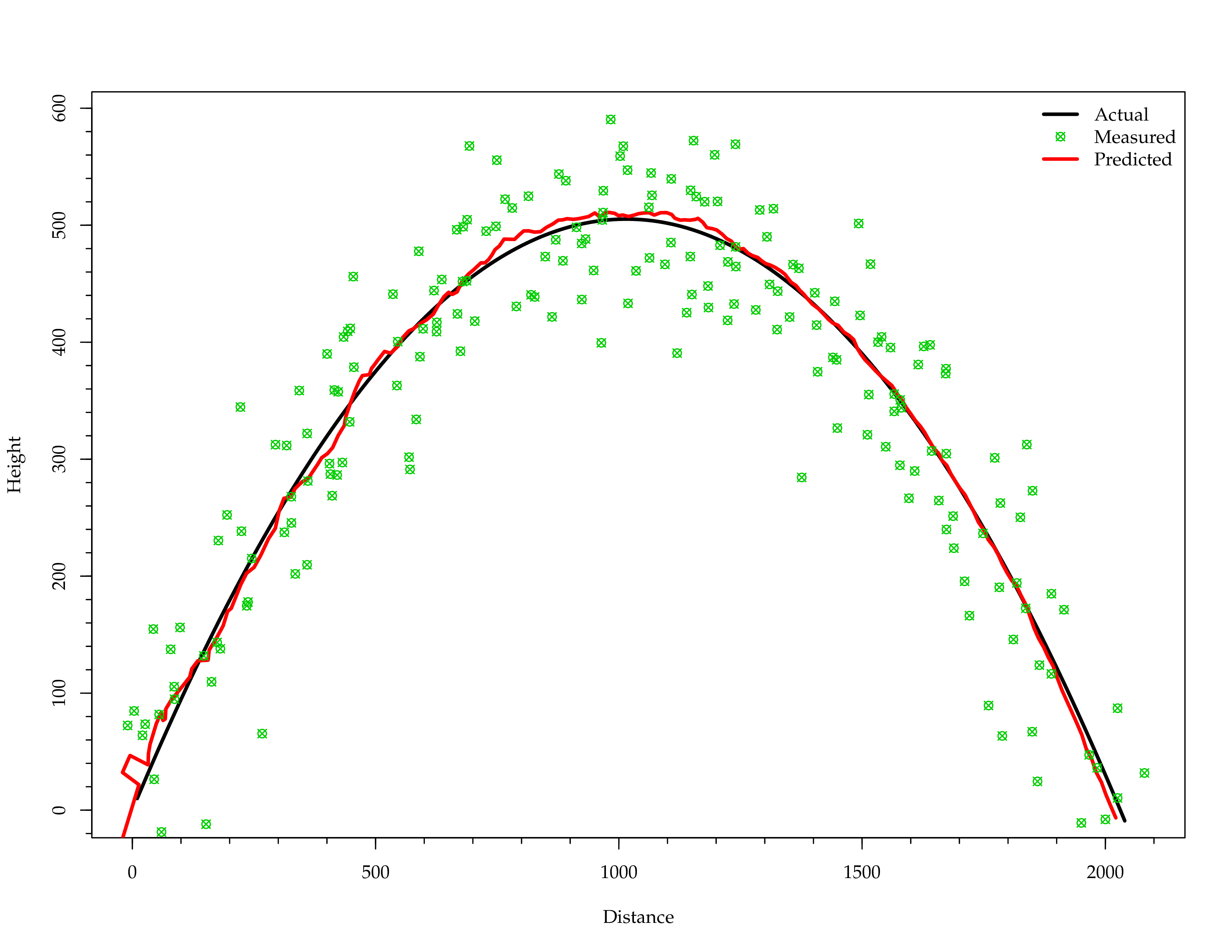# Example: PCA  val jpg = ImageIO.read(file("input.jpg")) val orig = imageToMatrix(jpg) val svd.SVD(u, s, v) = svd(orig) for { i <- 1 until u.cols if i <= 100 // no discernable difference beyond! } { val compressed = u(::, 0 until i) * diag(s(0 until i)) * v(0 until i, ::) val out = f"compressed-$i%03d.bmp"
val converted = matrixToImage(compressed)
ImageIO.write(converted, "BMP", file(out))
}

# Graphics Cards (GPU)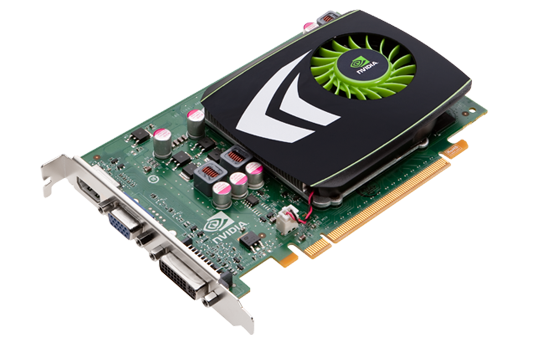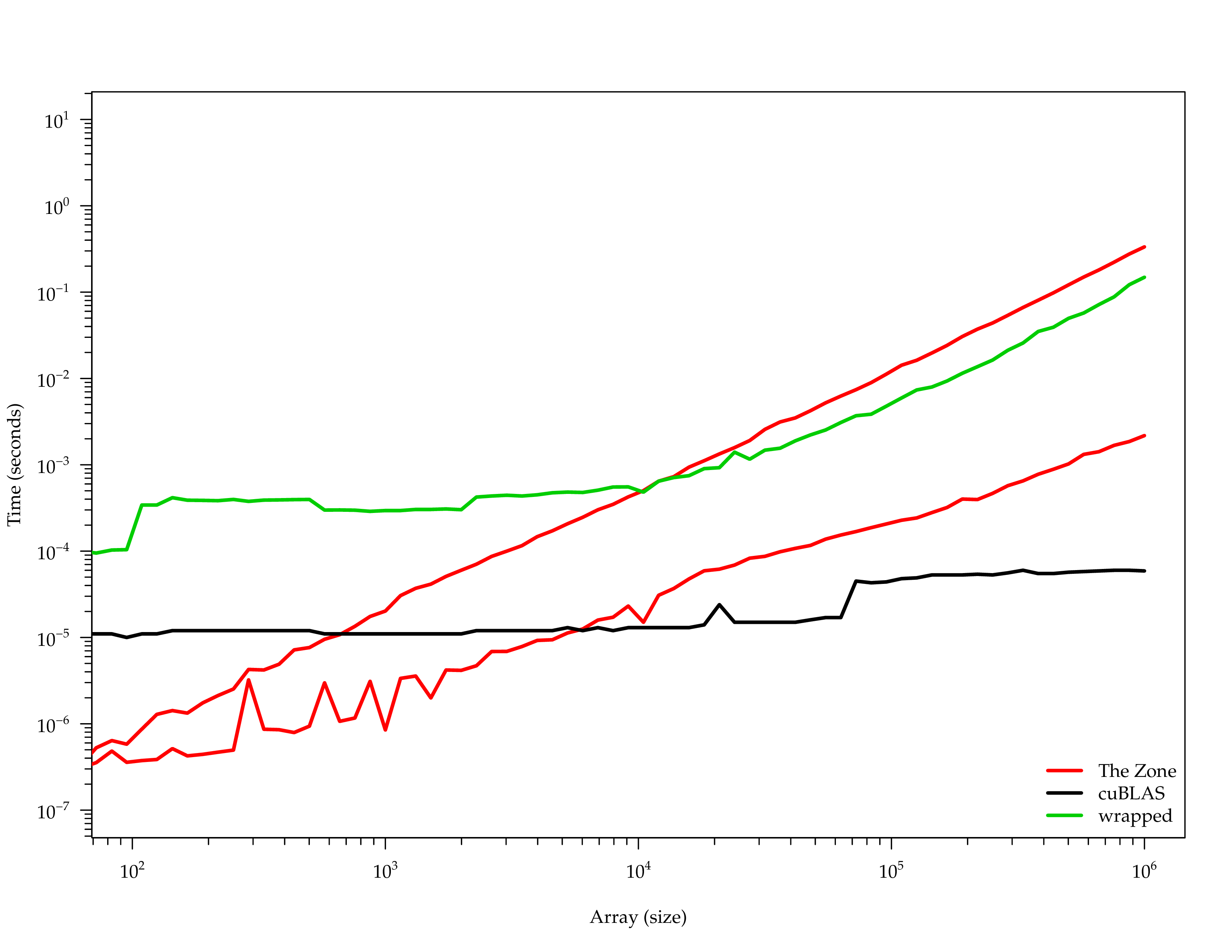# FPGAhttp://analyticsengines.com

# APU